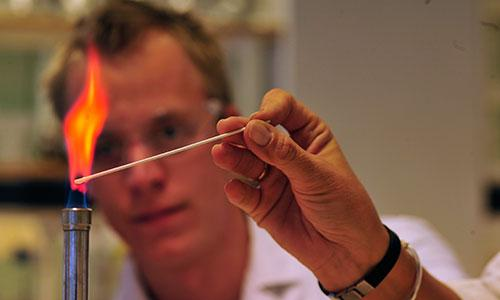# Are You A Master Of The Periodic Table?

10 Questions | Total Attempts: 550SettingsDo you think you are a master of the periodic table of elements? Take the quiz and find out!

Related Topics
• 1.
Fill In The Blank The periodic symbol for Iron is
• 2.
Choose the correct answer. What is the first periodic element in the upper left corner of the periodic table?
• A.

Calcium

• B.

Hydrogen

• C.

Helium

• D.

Lithium

• 3.
Fill In The BlankMg is the periodic symbol for
• 4.
True or False?The periodic symbol for Fluorine is Fr.
• A.

True

• B.

False

• 5.
Choose the correct answer.How many Alkali Metals are shown on the Periodic Table?
• A.

6

• B.

8

• C.

4

• D.

12

• 6.
Choose the correct answer.C is the periodic symbol for what element?
• A.

Copper

• B.

Calcium

• C.

Carbon

• D.

Chlorine

• 7.
True or False?Sulfur is located right below Oxygen on the periodic table.
• A.

True

• B.

False

• 8.
• A.

5

• B.

7

• C.

9

• D.

6

• 9.
True or False?There are no 3 letter periodic symbols.
• A.

True

• B.

False

• 10.
Choose the correct answer.What is the periodic symbol for Platinum?
• A.

Pl

• B.

Pt

• C.

Pn

• D.

Pa